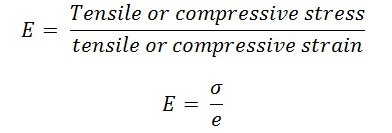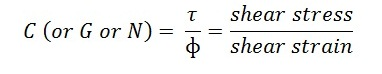# What is Elasticity, Elastic Limit, Young’s Modulus and Modulus of Rigidity in Strength of Materials?

In this article, we will learn about what is elasticity, elastic limit, young’s modulus and modulus of rigidity. These terms keeps an important role in the study of subject strength of materials. Understanding about stress and strain is possible when one must have the knowledge of these terms.

Let’s discuss about them one by one.### What is Elasticity?

When an external force is applied on a body and it undergoes some deformation. If the body returns back to its original shape and size on complete removal of the load, the body is called elastic body. This property by which any material regains its original shape and size when load acting on it is completely removed is called elasticity.

Elasticity: The property of a material by which it returns back to its original position (i.e. shape and size) on the removal of external force or load, is called elasticity.

### What is Elastic Limit?

A body will return back to its original shape and size when the deformation caused by the external force, is within certain limit. There is a limiting value of force upto and within which the deformation caused completely disappears on the removal of external force. The value of stress corresponding to this limiting force is known as elastic limit of the material.

Elastic Limit: It is defined as the value of stress upto and within which the material return back to their original position (i.e. shape and size) on the removal of external force.

If the value of external force is such that it exceeds the elastic limit, than the body will not completely regain its original position. The body loses its property of elasticity to some extent. And if the external force acting on the body is removed, in that condition the body will not return to its original shape and size and there will be a residual deformation in the material.

### What is Young’s Modulus?

Young’s Modulus: The ratio of tensile or compressive stress to the corresponding strain within elastic limit is called young’s modulus.

• Young’s modulus is also known as modulus of elasticity. It is
denoted by E.
• The formula of Young’s modulus is given byWhere,

σ = Tensile or compressive stress,

e = Tensile or compressive strain,

E = young’s modulus or modulus of elasticity.

### What is Modulus of Rigidity?

Modulus of Rigidity or Shear Modulus: It is defined as the ratio of shear stress  to the corresponding shear strain within elastic limit.

• It is denoted by C or G or N
• The formula of modulus of rigidity is given byWhere,

τ = Shear stress

ɸ = Shear stress

C = Modulus of rigidity

This is all about what is elasticity, elastic limit, young’s modulus and modulus of rigidity. If you find anything missing or incorrect than comment us. And if you like this article than like us on Facebook.